# Cake and Duplicates

Post Page Cover Image credit: The ProFroster. Fun fact: our team helped design and manufacture these!

Let me talk to you about cake. Hungry yet? Good. Hungry for knowledge?! Even better. Because I’m not talking about cake that you eat — I’m talking about Interview Cake.Let me lay out the problem for you.

## The Problem

The problem is this:

You are given a list of integers in the range (0, n] (excluding 0, including n), that fill an array such that there are n + 1 numbers in the array. In other words, this array has one of each integer except for one unknown value that has a duplicate. The numbers in the array are not in any particular order.

Example: [4, 3, 7, 1, 5, 3, 2, 6] : 8 numbers, 1..7. The only duplicate is 3.

If you’re feeling spunky, go ahead and give it a try. This is just the first portion of the problem, but it’ll help you get your head in the game.def find_duplicate(nums)
nums.select { |num| nums.count(num) > 1 }.first
end


Alright, that’s it. That’s the whole thing, article over. Just kidding. I forgot to mention:

## The Rest of the Problem

Here’s where it gets interesting: the last two requirements of the problem.

1. Your solution must be better than O(n^2) in time.
2. Your solution must remain O(1) in space.

To make sure everybody is on the same page with me in terms of what that means, let me do a 30 second explanation of “Big-O Notation”, which is what those O(n) and O(1) are called. If you’re already comfortable with Big-O, go ahead and skip ahead.

### Big-O Notation

“Big-O Notation” has to do with how efficient an algorithm or program is, as a function of the number of items used as inputs. Let me put it this way: Let’s say you’re given n numbers in your input list. If your solution requires you to loop through the whole list once (in the worst case, like if the number you’re looking for is at the end of the list), then your solution is considered O(n). O(n) is also known as linear efficiency. This is because, if we double the size of the input to 2*n, your algorithm will have to process twice as many steps and most likely will be twice as slow. If we increase the size to 100*n, your algorithm will similarly do 100*n steps.

Now, what if you had a function that was O(n^2)? That would mean that your function looped through each of the n inputs n times (e.g. in a double for-loop). Thus, if, instead of n inputs, you had 2*n inputs, your program would actually have to do (2*n)^2 or 4*n steps! If your program had 100*n inputs, it would have to do (100*n)^2 steps — 10000*n! Hopefully you can see that O(n) is quite a bit more efficient than O(n^2).

So what does O(1) mean? The 1 is a little misleading, because it simply stands for constant time, meaning that no matter how many inputs you have, your program will always take the same amount of steps, and thus, roughly the same amount of time. This is the Holy Grail of algorithms. This is the reason why it’s so much faster to look up items from a Hash (or dict, or object, or whatever your language of choice calls it) than it is from a List or Array.

The last thing: Space Complexity. Space Complexity works the same as Time Complexity, except it has to do with how much computer memory you’re using. Some examples might get things accross better.

O(1): When you have 10 inputs, your program keeps track of 3 variables. When you have 20,000 inputs, your program keeps track of 3 variables.

O(n): When you have 10 inputs, your program keeps track of two separate extra lists for a total of 20 slots. When you have 20,000 inputs, you need 40,000 extra slots to keep track of everything.

O(n^2): When you have 10 inputs, you need 100 extra slots. (Don’t ask me why, you wrote the program. Seems like overkill). When you have 20,000 inputs, you end up needing 400,000,000 extra slots (and also a new computer because, congratulations, you killed this one).

Hopefully this super brief explanation helps. If you’re still confused, Vaidehi Joshi does a better job than me of explaining it, with pictures! See also the whole BaseCS series. See also also the BaseCS Podcast.

ONWARD!

### Rejecting Some Possible Solutions

#### Possibility 1: The Naive Solution (a.k.a. My Solution)

So what do those requirements really mean for us? Well, let’s look back at my initial solution. For each item in the list, I go back through the list again and count the number of occurrences. My function is actually O(n^2) in Time (a double-for-loop!).While you might argue that my solution is “good enough” for most cases (and I would agree), that’s not what the requirements say we have to do.

#### Possibility 2: The Other Naive Solution (a.k.a. Keeping Track)

You could loop through just once, keeping track of the numbers you’ve already seen in a set. Once you see a number that’s already in your set, you know that’s the duplicate!

require 'set'

def find_dupes(nums)
history = Set.new
nums.each do |num|
return num if history.include?(num)

history << num
end

return -1  # We should never reach this stage
end


How’d we do? Well, we only ever loop through the whole list once at most, bringing us to O(n) Time Complexity. Right on! But we create the history set that could contain (in the worst case) almost all n of our input values. This looks like O(n) in Space as well, which means that we fail the second requirement. 💩!

#### Possibility 3: The Sorting Solution (a.k.a. The Slightly Tricky Solution)

What about doing a sort? Since sorting can be done in O(nlogn) time, which is better than O(n^2), we might have a chance. We could sort our numbers, and then loop through them once while only keeping track of the last number that we saw. If we see the same number twice, it’s a duplicate.

def find_dupes(nums)
nums.sort!
previous = 0
nums.each do |num|
return num if num == previous

previous = num
end

return -1
end


This gets us O(1) in Space and O(nlogn) (ish) in Time. One problem with this. If we don’t create a new array to put our sorted numbers in, we’re destroying/modifying the order of the list that gets passed into us, which isn’t good. If we create a new array to put our sorted numbers in, we jump up to O(n) in Space, which is a fail. Hmmm… There’s a better way — trust me.

## The Better Way

OK. Here’s the solution they provided. It has some CS concepts in it, but don’t worry. I’ll walk you through. I don’t think I would have ever thought of it in a million years, but I guess that’s why we practice, right?

### A Linked List (Sort Of)

OK. Do me a favor and imagine the input list as a series of connected nodes: a Linked List, if you will. Each slot in the array has its own index and the number that points to the index of another node in the list. The original problem on Interview Cake bases things on a 1-base array, so that’s how I’ll explain things too. Thus, we end up with something like this: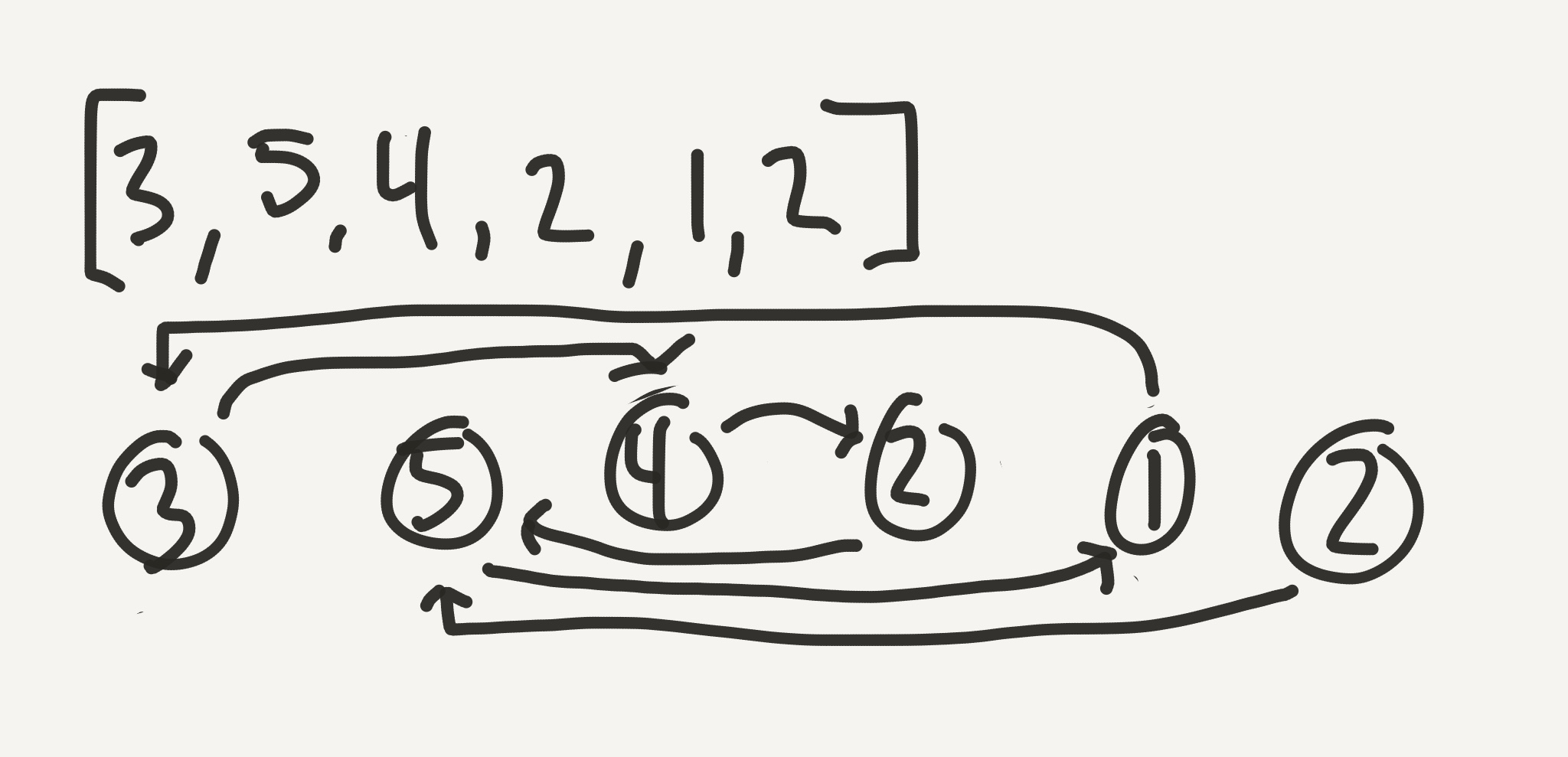Study that list for a minute. Get a piece of paper and pencil and try some more. [3, 1, 2, 4, 1]. [8, 6, 4, 2, 1, 2, 5, 3, 7]. [2, 1, 1, 3]. What do those look like? Do you start to see patterns? I mean, besides the pattern that I suck at freehand diagrams?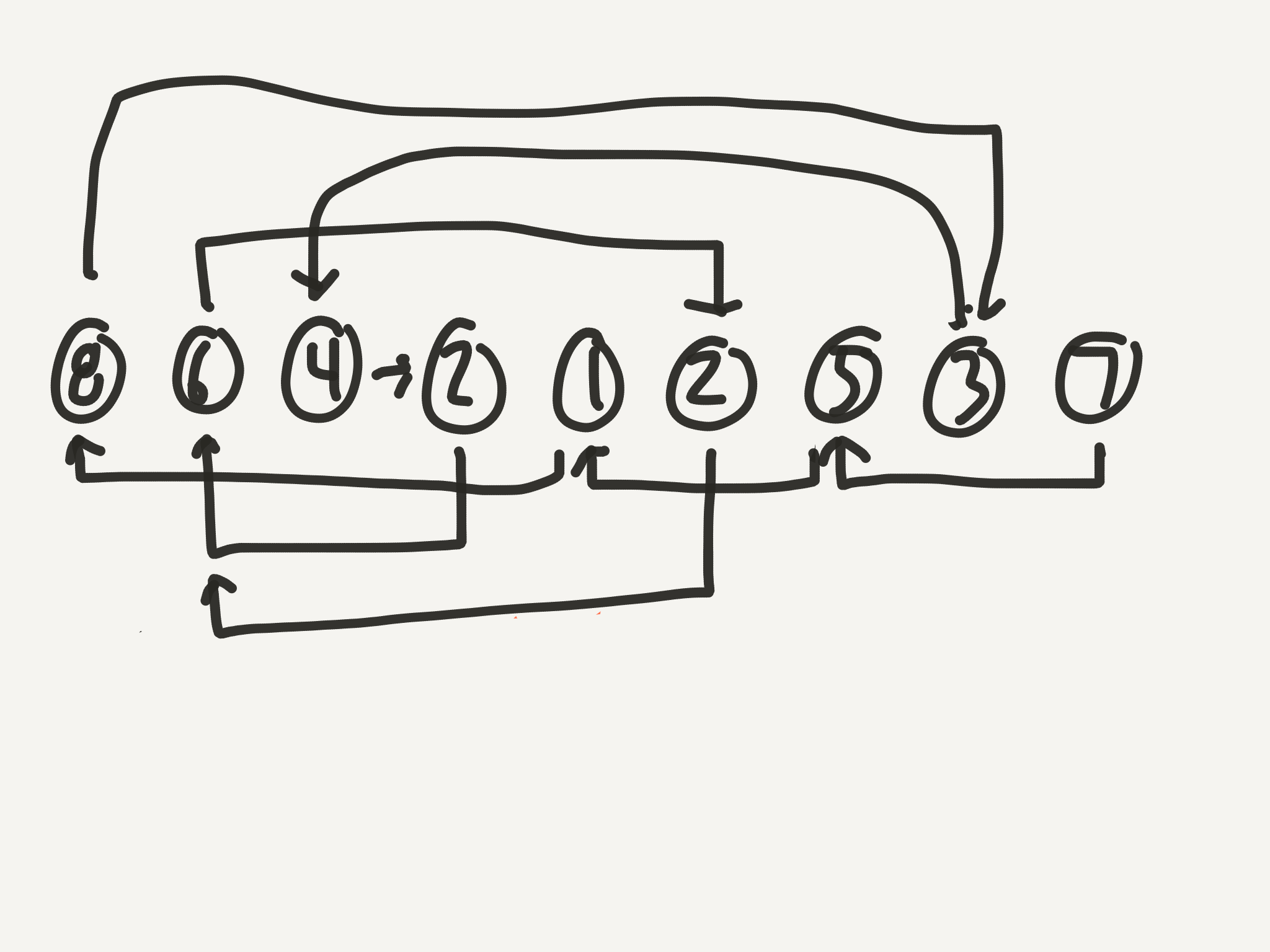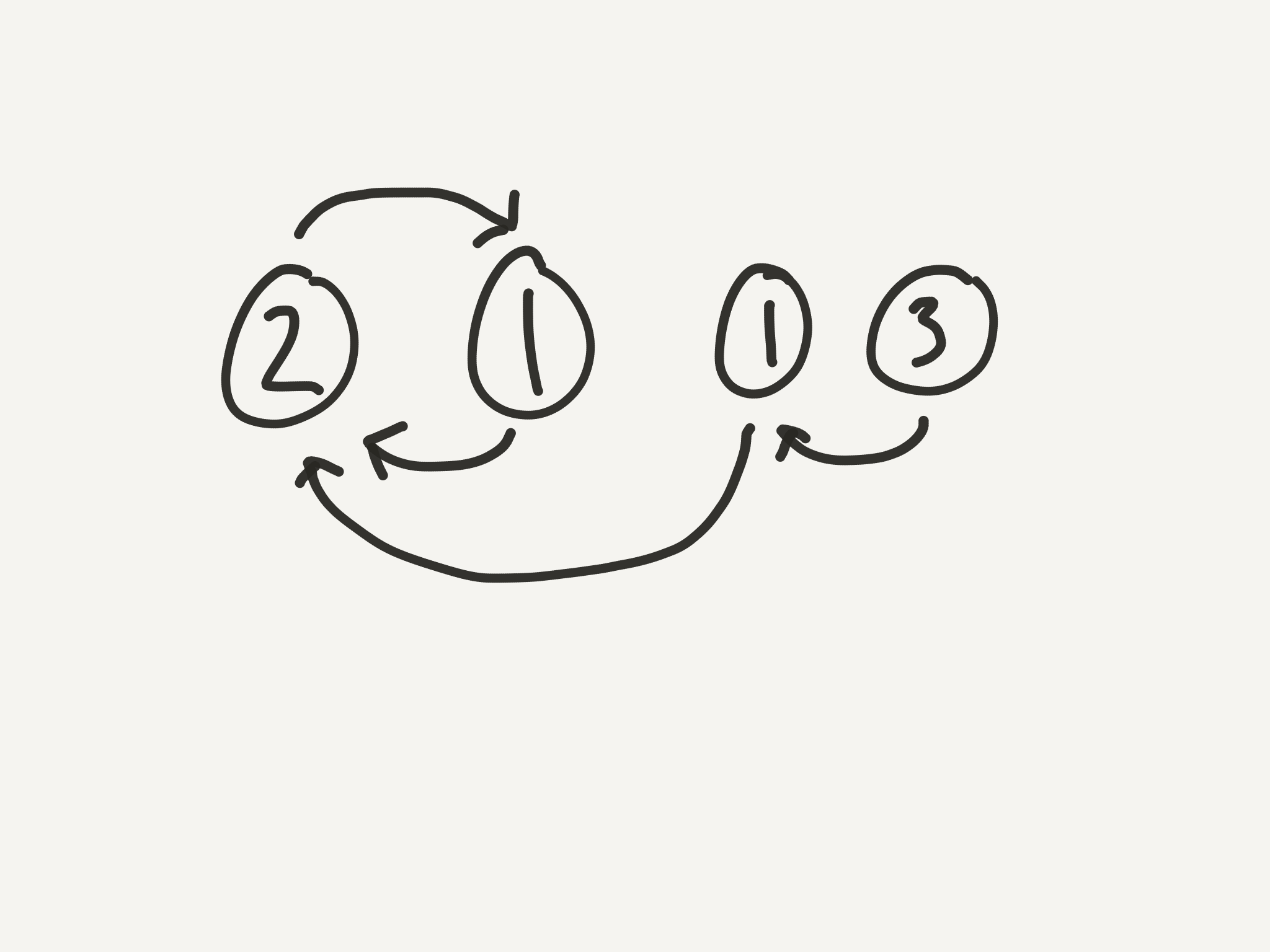Here’s some things to note:

1. The last node never has any arrows pointing at it. That’s because it’s at position n+1, and the maximum value in the list is n. If we’re thinking of our array like a kind of linked list, the last node would be a good candidate for the head.
2. Because there is always a duplicate, there is always a loop somewhere in the list. At some point, every diagram becomes cyclic.
3. Because there is always a cycle, there is always a node that has two arrows pointing at it.
4. This node with two arrows pointing at it is always the first node in the cycle.
5. The index (1-based) of this two-arrow node is always the duplicate value.So how does that help us? Well, if we can find a way to figure out where the start of the cycle is, we can return the index of that node as our result.

### Finding the Length of the Cycle

To find the start of the cycle, we’ll need its length. Trust me on this, and it’ll make sense in a little bit. That is actually not as hard as you might think. In order to get the cycle’s length, we need a sure-fire way to get into the cycle. Well, we only have a certain number of nodes, right? So, if we just start stepping, we might end up in the cycle at some point, but after n+1 steps, we’re guaranteed to be somewhere within the cycle. Because, even if the cycle is the size of the entire list like this: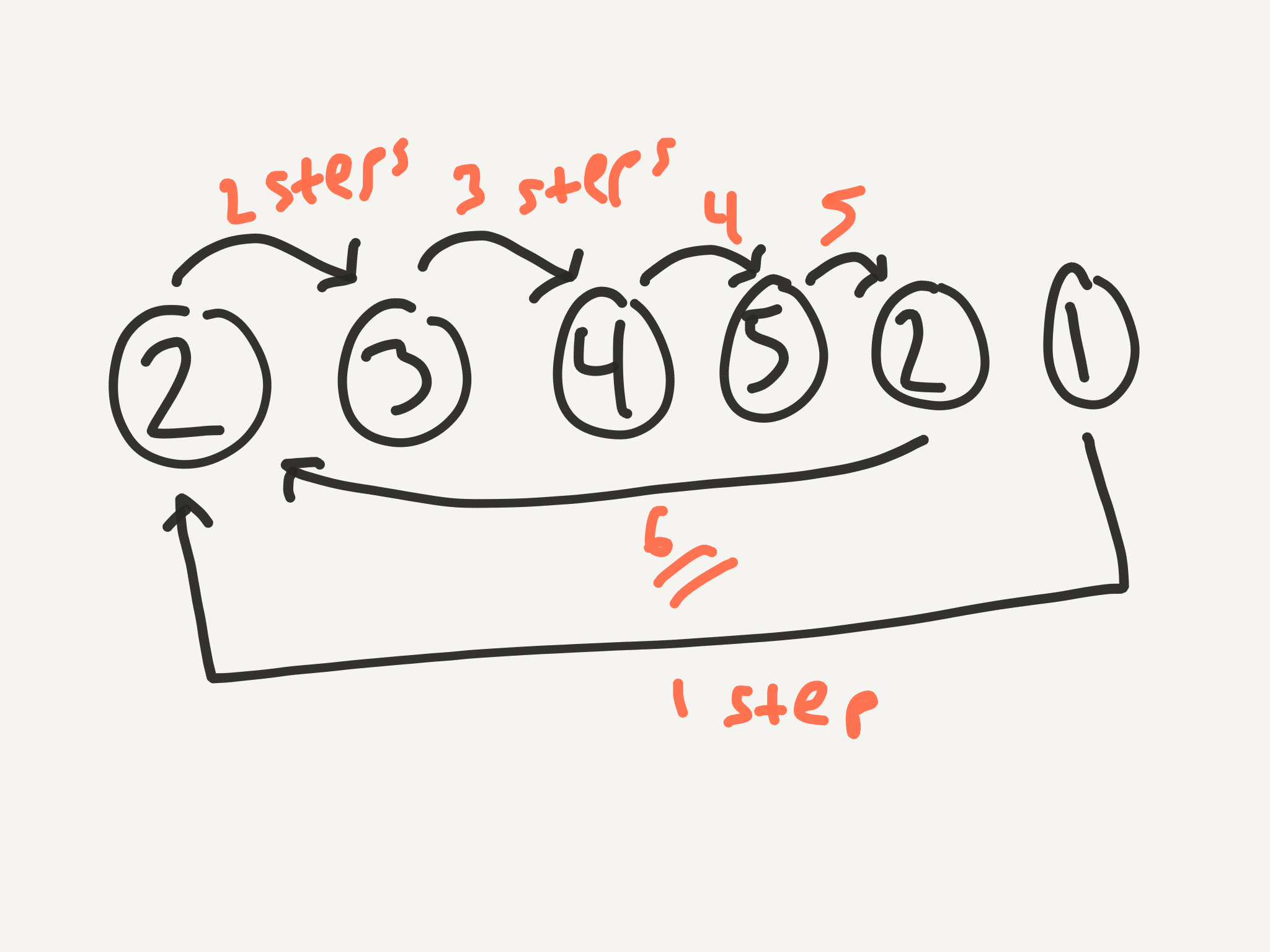You still can only go to each of the nodes once before you create a cycle. I’ll try to show my solution being built as we go. If you’re somebody who has to see things written out in code, hopefully this will help you.

class DuplicateFinder
def initialize(nums)
@nums = nums
end

def step(current)
# Convenience method to move to next node
@nums[current - 1]  # -1 because of 1-based counting
end

def find_a_number_in_the_cycle
next_step = @nums.last
@nums.length.times do
next_step = step(next_step)
end
next_step  # Returns the number at the index we're on
end
end


OK. So now we’re in the cycle, so what do we do? Well, within the cycle, the numbers are all unique! So all we have to do is remember the node we’re at initially, and start stepping and counting. Once we see the same number again, we’ll know that we’ve travelled the loop exactly one time and we’ll know how long the loop is!

class DuplicateFinder
# ...

def length_of_cycle
stop_num = find_a_number_in_the_cycle
next_num = step(stop_num)
steps = 1
until next_num == stop_num
next_num = step(next_num)
steps += 1
end
steps
end


### The Yardstick Method

Now we’re at the real amazing part. It has to do with something that could be called the Yardstick Method (or, I suppose, the Meterstick Method, if you must 😉). Let’s go back to the head of the list and start over. For example. Let’s say we have the list [4, 1, 2, 3, 4, 5, 6, 7, 8, 9]. If you draw it out, the cycle is 4 nodes long. So, what we need to do is imagine we have a stick that is 4 nodes long, just like the cycle. We can translate this into code by using two pointers: one at the head of the stick and one at the tail.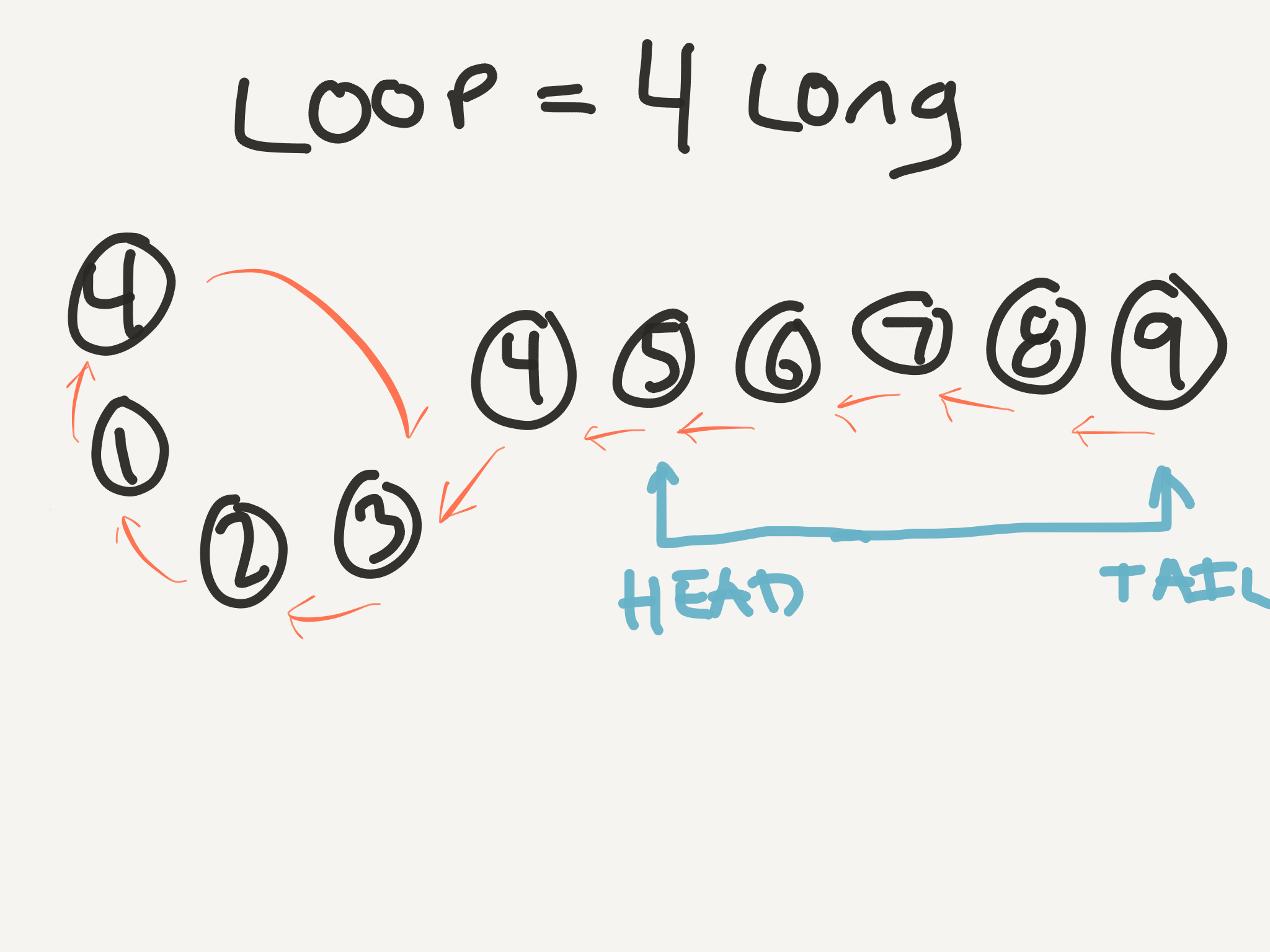Now we step our whole stick forward one node, moving both the head and the tail, so the stick stays the same length.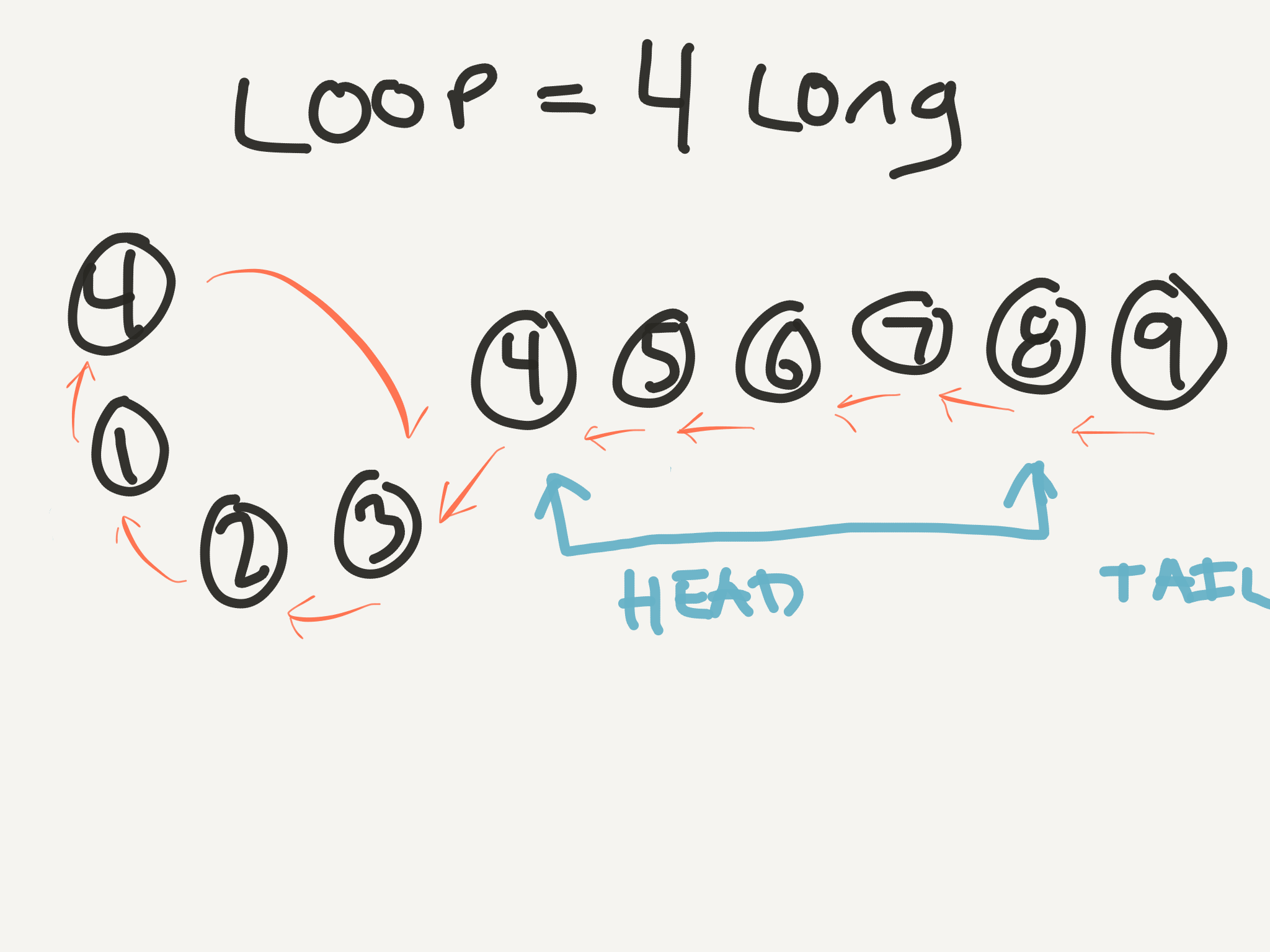What happens if we continue this process?Both the head and the tail of the stick are pointing at the same number! And it’s the number with two arrows at the beginning of the cycle! AND what index (1-based, like our linked list) do they meet at? Index 4! AAAAND what is the duplicate number in our list? 4!For completeness, how does this look in code?

class DuplicateFinder
def initialize(nums)
@nums = nums
end

def step(current)
@nums[current - 1]
end

def find_a_number_in_the_cycle
next_step = @nums.last
@nums.length.times do
next_step = step(next_step)
end
next_step
end

def length_of_cycle
stop_num = find_a_number_in_the_cycle
next_num = step(stop_num)
steps = 1
until next_num == stop_num
next_num = step(next_num)
steps += 1
end
steps
end

def duplicate
stick = length_of_cycle
tail = @nums.last
tail = step(tail)
end
# When head and tail point to the same number
# then on the next step, they'll be on the same node
# This current same number means head and tail both = the duplicate!
end
end


## Evaluation

So, how’d we do?

Space: We generally keep track of the next node to step to, and a few other variables, but I think we can safely say that our space usage is ~O(1). We don’t ever save a copy of our array anywhere.

Time: We loop through once to find the length of the cycle, and then we loop again to find the duplicate. This gets us to ~O(n) since we always loop through the array the same number of times regardless of input length and it’s only a matter of how long the array is.

VICTORY. This was a really tricky one. If it didn’t make sense, it’s not your fault. I had to read through it a few times, do the problem a few times, and even write this blog post before I fully understood how it worked. Feel free to reach out with questions if I can help clarify anything for you. And again, go check out Interview Cake for more practice on puzzles like these (although they’re not all quite as tricky as this one is).

Author: Ryan Palo | Tags: algorithms ruby big-o linked-lists |

Like my stuff? Have questions or feedback for me? Want to mentor me or get my help with something? Get in touch! To stay updated, subscribe via RSS